# Clever choices for optimization

Abstract This tutorial is based on a master class on fast LC. It provides an insight into the effect of parameter changes in fast LC, e.g. the influence of flow, pressure, temperature and particles size. It is supported on a solid theoretical background. Together with many application results of the various columns and parameters it gives a good guidance in how to optimize your analysis. This chapter will help you:

To learn and understand the rules of thumb
To find the balance between time and separation.

There are several interpretations of the term Fast LC.

• “Easy separation HPLC”
• “High throughput HPLC”
• “Perform my separation in shortest possible time”

We will focus in this master class on the last option: finding the shortest possible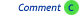separation time for a given number of plates.

There are several parameter we can change. We will discuss here the following options:

• Change particle size?
• Switch to monolith?
• Change column temperature?
• Change column pressure?
• Change column length?

## Rules of thumb

As a general rule of chromatography we can state: if a separation problem is difficult, you will have to cope with a large analysis time.

So we need to be clever when we optimize. Here are the essential rules of thumb that can be learned from this chapter:

• First: optimize your selectivity
• If Fast HPLC = Easy separation HPLC, then use small N, short L, small dp, and high pressure
• If you achieve the desired resolution well below the P-limit: then reduce dp or increase L until you reach P-limit
• If you achieve desired resolution near the P-limit: no need to change L or dp,change in P might help.
• Be aware of ratio extra-column over column volume: favours use of larger particles
• Temperature always helps to speed up separations, provided retention capacity can be kept sufficiently high
• Optimization of column length can already yield a significant increase in analysis speed or separation efficiency.

• To understand these rules of thumb in depth
• To optimize the analysis
• Find the balance between time and separation.

We will start with some tricks to speed up your analysis.

## Tricks to speed up your analysis

In this part we will play with the various parameters, to give you a sense of their effect. We developed for this reason a chromatogram generator. After filling in your parameters the generator will produce the chromatogram. Use this link to go to the article where you can download the generator. We advise you to use it to reproduce the values in this tutorial and also play with the values yourself.

We will now give some examples in which we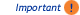vary the following parameters:

• Higher T
• Smaller particles
• Smaller particles and shorter column
• Smaller particles and shorter column and higher pressure
• Smaller particles, shorter column, higher pressure and T
• Back to 30 °C

Higher T
On the example left you see our reference example. On the the right we have increased the temperature to 90oC. The resulting chromatogram at higher T shows a faster analysis with similar resolution.
Click on the specific figure to see the full chromatogram, we advice to use the chromatogram generator yourself (which we prefer, since we think playing with the generator gives you a better feel of how the parameter influence the result).

Smaller particles
Next we show the effect with smaller particles, changing to 1,7µm. This leads to a higher analysis time. When we now decrease the column size to 100 mm, we see an improvement in speed of analysis. Click on the specific figure to pop up full chromatogram.When we now also increase the pressure to 1000 bar, we see a great improvement in analysis time (left), and on the right we have increased the temperature to 120oC.

## Change particle size?

Option: particle size.
You could choose for instance between sub-2 or 3.5 µm particles.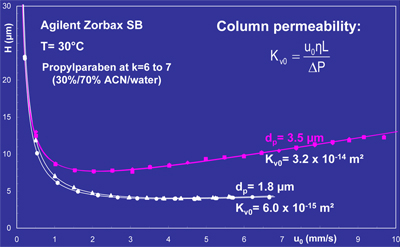Which particle size ispreferred?

To determine the desired N we can use the kinetic plot method. The formula to determine the N is:We can calculate by putting this formula in an Excel spreadsheet the H as a function of flow with different particle sizes: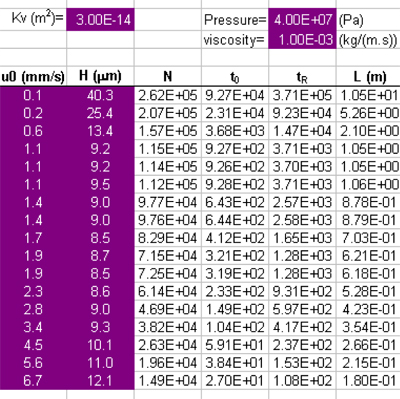Starting from a set of experimental data (just two columns), a whole list of optimal combinations between N and time is obtained.

If we plot these data in graphs of tR versus N (below) we can readily see that:

• If you require a small N or a low peak capacity: use small particles
• If you require a large N or a large peak capacity: use large particles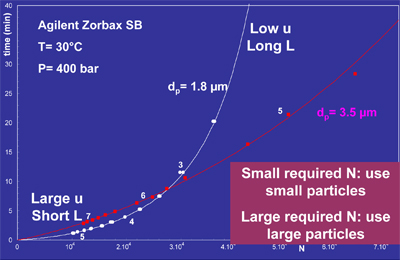## Optimal particle size

General rule of thumb:

• Select dp and L such that you achieve desired N at µ=µopt and ΔP= ΔPmax

For example: if you reach the desired N while ΔP << ΔPmax , then switch to a column with smaller dp
To understand this better, let us first look at the influence of the particle size on the plate height curve and the column permeability: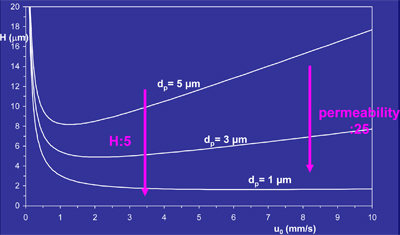Roughly speaking, decreasing the particle size five-fold results in a five-fold reduction of H (around the curve minimum), but also in a 25-fold (!) reduction of the column permeability. This leads to the following set of kinetic plot curves:• The ideal region where we would like to see our columns operate is of course in the right, downmost corner. There N is largest and the time is shortest. As can be seen: each type of  particle size gets closest to the right downmost  corner when the maximal pressure is reached when µ = µopt
• The line connecting these different optimal points is the so-called Knox and Saleem-limit (see dashed pink line. )
• Supports with a given shape (e.g. well packed spheres) can never offer an operating point that lies on the right hand side of this line.

By changing the particle size, one can move the optimal operating point along this line, as indicated by the turquoise arrow in below figure: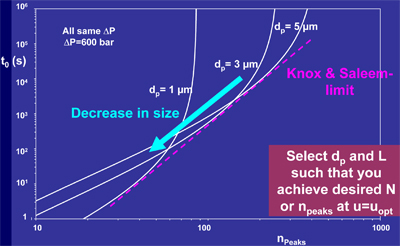## Optimal column pressure

Rule of thumb:

• A large P is most effective for applications that require a large N.

To make this clear we start out with some examples:

400 bar600 bar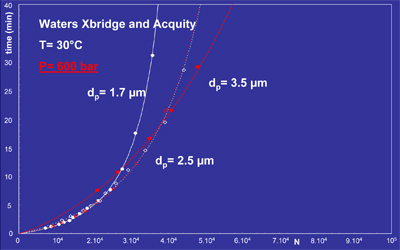1000 bar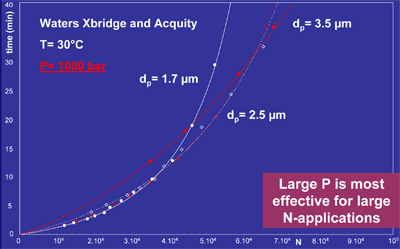The decrease in analysis time that can be observed when comparing the three shown pressure cases is, especially in absolute terms, most pronounced in the large N region. This shows that a large pressure is especially needed if you have to solve different separation problems. If your separation only needs 10,000 pates, the gain you obtain by using pressures above 400 or 600 bar is really small (in the order of 1 minutes or less).

Band broadening changes with particle size.
Important rules of thumb are here:

• Fast HPLC requires minimal system volumes
• Large system volumes favour the use of large dp.

Measurements of extra-column band broadening are very tedious and often lead to highly scattered measurements, as can be seen in the example below: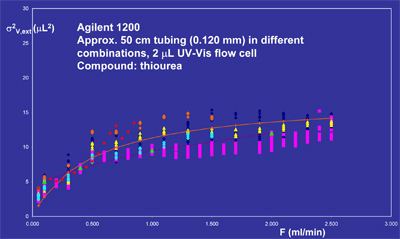This large scatter is probably the reason why the extra-column band broadening is poorly studied. This is a pity, because this extra-column band broadening can have a dramatic effect on the kinetic performance and even on the optimal particle size.

This is shown in below series of curves, where, for a fixed extra-column system, different column diameters are considered.

In the first figure, the extra column band broadening effects were excluded, given the expected behaviors: large dp is good for large N-separations, while a small dp is best for small separations.When the extra-column band broadening is taken into account, this general picture does not change much when 4.6 µm columns are being used: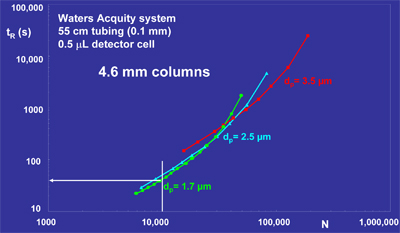However, when passing to 2,1 and 1 µm columns, the effect of the extra-column band broadening becomes more apparent, eventually leading to a situation (in 1 µm columns), wherein 1,7 um particles no longer have any range of applications wherein they are advantageous.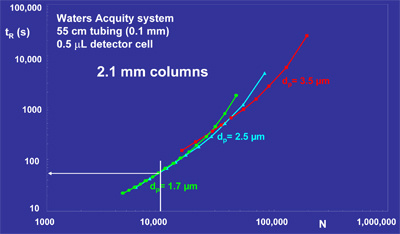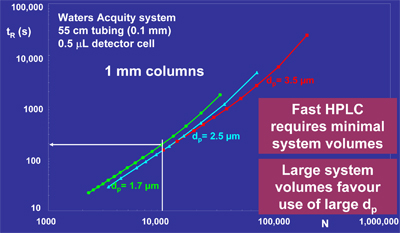## Switch to monolith?

Rule of thumb:

• Current silica monoliths have too large pore sizes.

Here are some examples to support this statement: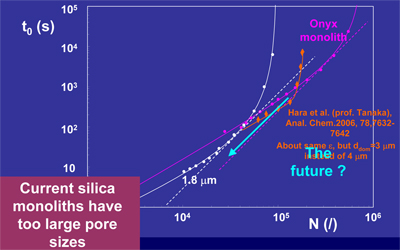## Column length?

This is an often overlooked parameter, but it has in fact very powerful optimization possibilities!

Rules of thumb:

• To avoid wasting your time: always work at ΔPmax!
• If you’re not operating at ΔPmax: switch to smaller particles or longer columns
• If I don’t work at this maximal pressure I am wasting my time

To understand this, let us first have a look at how tand N vary in a set of columns with different lengths as a function of changing pressure (see red arrow in below two figures).Overlapping this set of curves with the kinetic plot curve (see white curve), we see that all the best possible operating points (i.e. the most righthand downmost situated operating points.). All fall on the kinetic plot curve, which is also the curve where everywhere ΔP = ΔP max.

This means that the only condition that needs to be satisfied in order to avoid costing your time is to work at maximal pressure.Of course, one also has to select the appropriate column length (i.e. the one that gives the desired plate number at a flow rate yielding ΔP = ΔPmax). Very often, this optimal column length is larger than the olumn length you are normally using.

Below example shows that if you are working at optimal flow rate in a 10 or a 20 cm  column  (operating point indicated by the crosses), you can always obtain a much higher efficiency or a much shorter analysis time by switching to a longer column (see red arrows).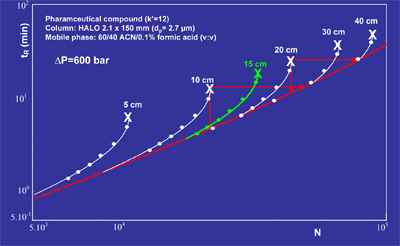This is illustrated in an example, showing that both N can be increased and t can be decreased by going to a longer(!) column.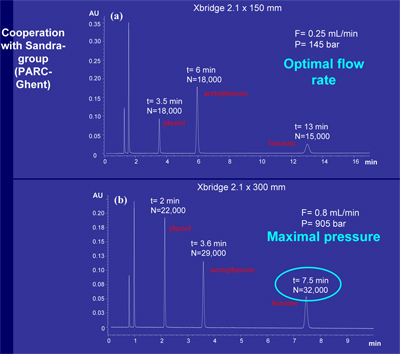The above also shows that on a lot of analysis time can be gained if one could tune the column length needed to achieve the required plate number at the flow rate for which ΔP=ΔPmax

This requires having the possibility to easily switch between different column lengths.

So, if you work for a rich employer, you could try to propose him the following system:

## The rich man's chromatograph## Effect of temperature

Measuring van Deemter curves at different temperatures leads to lower C-term band broadening, but also to a marked reduction of the viscosity of the mobile phase. This allows to use higher flow rates, and/or longer columns.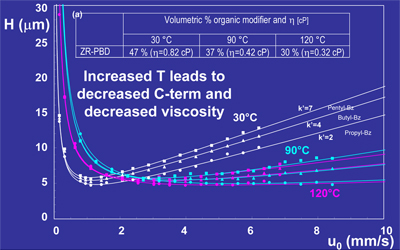Again, the combined effect can best be visualized in a kinetic plot: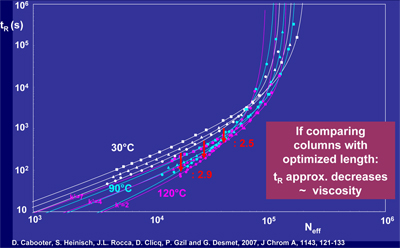A first conclusion of this plot is that temperature can not be used to increase the maximal efficiency one an obtain (this is different from the use of high pressures, where a high P is most effective in a high N-range.)

Another conclusion is that, in the small N-range, the reduction in analysis time one can expect when comparing an optimized length system at 30 oC  and an optimized length system at 80 or 120 oC is roughly proportional to the decrease in viscosity.

For the present case, this is roughly a factor of 2-3.

Claims about gains in analysis times of the order of 10 or more are only possible if comparing a non-optimized system at low T with a fully optimized system at high T.